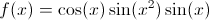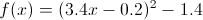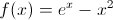# How to Solve Equations by Graphing on the CLEP Scientific Calculator - Quiz & Worksheet

Instructions:

question 1 of 3

### For these questions, I strongly recommend using either the CLEP calculator (found online) or one you use in class. Where is the maximum value of f(x) between 0 and pi?Create Your Account To Take This Quiz

As a member, you'll also get unlimited access to over 84,000 lessons in math, English, science, history, and more. Plus, get practice tests, quizzes, and personalized coaching to help you succeed.

Try it risk-free for 30 days. Cancel anytime

### 1. For these questions, I strongly recommend using either the CLEP calculator (found online) or one you use in class. At what is the minimum value of f(x) between 0 and 1?### 2. For these questions, I strongly recommend using either the CLEP calculator (found online) or one you use in class. What is the root of f(x)? (where f(x) is equal to zero)Create your account to access this entire worksheet
Quizzes, practice exams & worksheets
Certificate of Completion
Create an account to get started

Utilize the graphing function on a scientific calculator to solve various equations. This quiz and worksheet will help strengthen your ability to solve these equations and the corresponding lesson walks you through the steps to solve the function.

## Quiz & Worksheet Goals

This quiz will assess your proficiency in solving for:

• Maximum values
• Minimum values
• Zero values
• Intersections

## Skills Practiced

This combination of quiz and worksheet allows students to test the following skills:

• Problem solving - use acquired knowledge to solve practice problems involving graphing functions
• Interpreting information - verify that you can read information regarding solving equations by graphing on a scientific calculator and interpret it correctly
• Knowledge application - use your knowledge to answer questions about minimum and maximum values, intersections and graphs
• Reading comprehension - ensure that you draw the most important information from the related lesson on solving equations by graphing on a scientific calculator

For more information on solving equation using a scientific calculator, review the accompanying lesson called How to Solve Equations by Graphing on a Scientific Calculator. This lesson covers the following objectives:

• Define features of a graph, such as minimum and maximum values
• Use a scientific calculator to graph single and multiple functions
• Identify zero values by graphing on a scientific calculator
• Find intersections between functions on a graph using a scientific calculator
Final ExamCLEP Precalculus: Study Guide & Test Prep
Status: Not Started
Chapter ExamUsing Scientific Calculators
Status: Not Started

Support